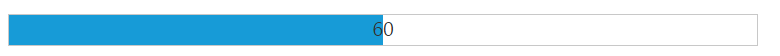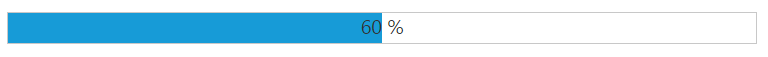# Define value

7 Jun 20172 minutes to read

## Value

The value for the ProgressBar is set by using value property. The value should be between the minimum (min) and the maximum (max) values (number) of the ProgressBar. By default, the minValue is 0 and the maxValue is 100 in ProgressBar, and the ‘value’ is set to 0(number).

The following code helps you that how to set the value for the ProgressBar widget.

• HTML
• ``````<div class="control">
<ej-progressbar id="progressBar" [minValue]="minvalue" [maxValue]="maxvalue" [value]="val" [height]="height" [width]="width" (create)="onCreate(\$event)"></ej-progressbar>
</div>``````
• HTML
• ``````import {Component} from '@angular/core';
@Component({
selector: 'sd-home',
templateUrl: 'app/components/progressbar/progressbar.component.html'
})
export class ProgressBarComponent {
minvalue: number;
maxvalue: number;
val: number;
height: number;
width: number;
constructor() {
this.minvalue = 40;
this.maxvalue = 80;
this.val = 60;
this.height = 20;
this.width = 500;
}
onCreate(\$event) {
var progress = \$("#progressBar").data("ejProgressBar");
progress.setModel({ text: progress.getValue() });
}
}``````

The following screenshot displays the output for the above code.## Percentage

The ProgressBar value is set in ProgressBar by using the ‘percentage’ property. The value range should be between the min and max values (number) of the ProgressBar. By default, the minValue is 0 and the maxValue is 100 in ProgressBar, and percentage is set to 0 (number).

The following helps that how to set the value in percentage for the ProgressBar widget.

• HTML
• ``````<div class="control">
<ej-progressbar id="progressBar" [minValue]="minvalue" [maxValue]="maxvalue" [value]="val" [height]="height" [width]="width" (create)="onCreate(\$event)"></ej-progressbar>
</div>``````
• HTML
• ``````import {Component} from '@angular/core';
@Component({
selector: 'sd-home',
templateUrl: 'app/components/progressbar/progressbar.component.html'
})
export class ProgressBarComponent {
minvalue: number;
maxvalue: number;
val: number;
height: number;
width: number;
constructor() {
this.minvalue = 40;
this.maxvalue = 80;
this.val = 60;
this.height = 20;
this.width = 500;
}
onCreate(\$event) {
var progress = \$("#progressBar").data("ejProgressBar");
progress.setModel({ text: progress.getValue() + " %" });
}
}``````

The following screenshot displays the output.